Molar Relationships Molar Relationships A balanced chemical equation

• Slides: 13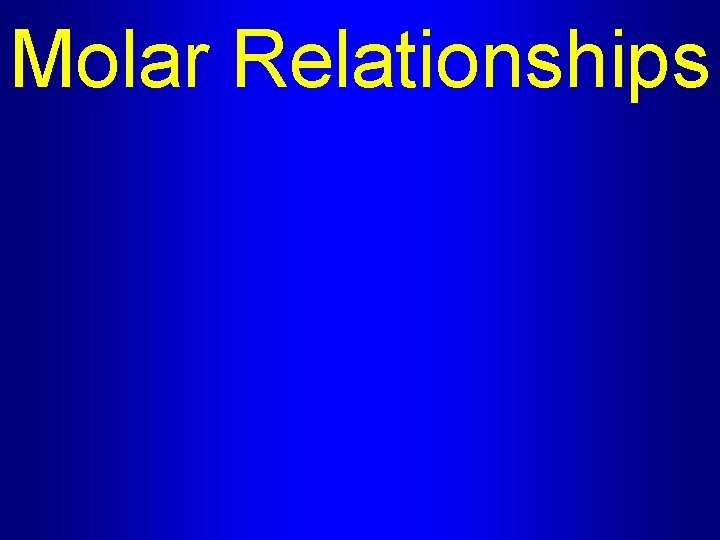Molar Relationships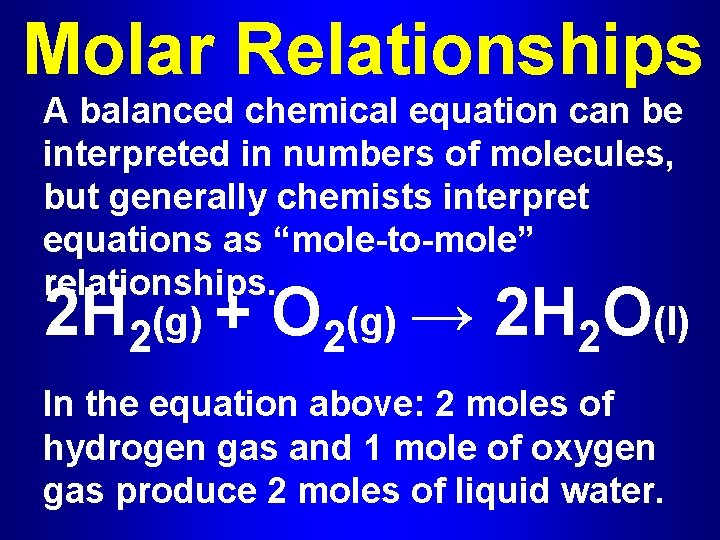Molar Relationships A balanced chemical equation can be interpreted in numbers of molecules, but generally chemists interpret equations as “mole-to-mole” relationships. 2 H 2(g) + O 2(g) → 2 H 2 O(l) In the equation above: 2 moles of hydrogen gas and 1 mole of oxygen gas produce 2 moles of liquid water.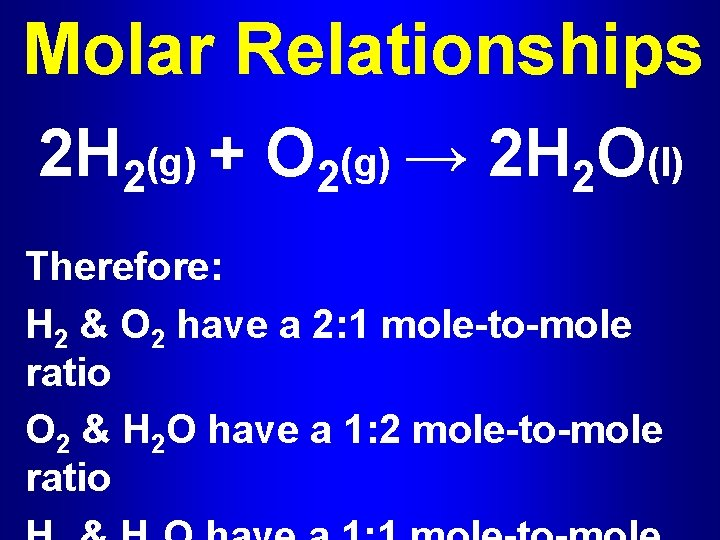Molar Relationships 2 H 2(g) + O 2(g) → 2 H 2 O(l) Therefore: H 2 & O 2 have a 2: 1 mole-to-mole ratio O 2 & H 2 O have a 1: 2 mole-to-mole ratio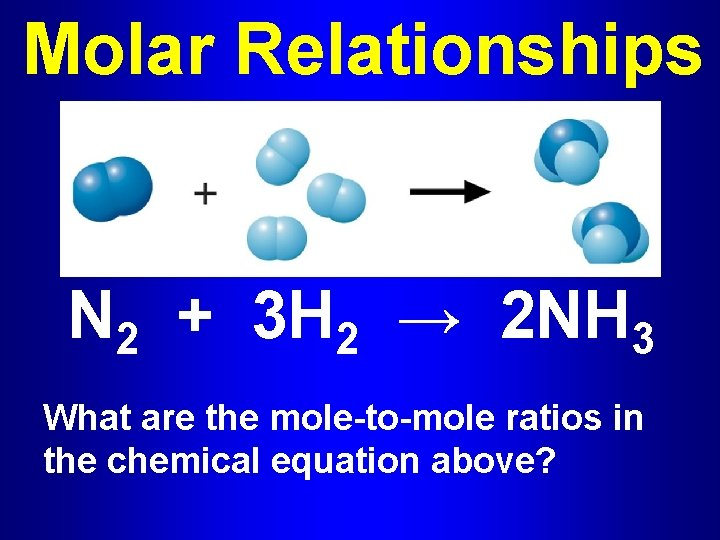Molar Relationships N 2 + 3 H 2 → 2 NH 3 What are the mole-to-mole ratios in the chemical equation above?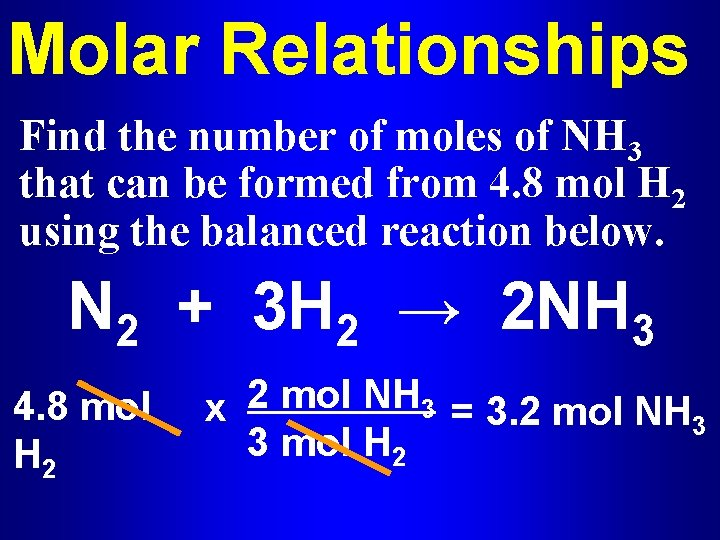Molar Relationships Find the number of moles of NH 3 that can be formed from 4. 8 mol H 2 using the balanced reaction below. N 2 + 3 H 2 → 2 NH 3 4. 8 mol H 2 x 2 mol NH 3 = 3. 2 mol NH 3 3 mol H 2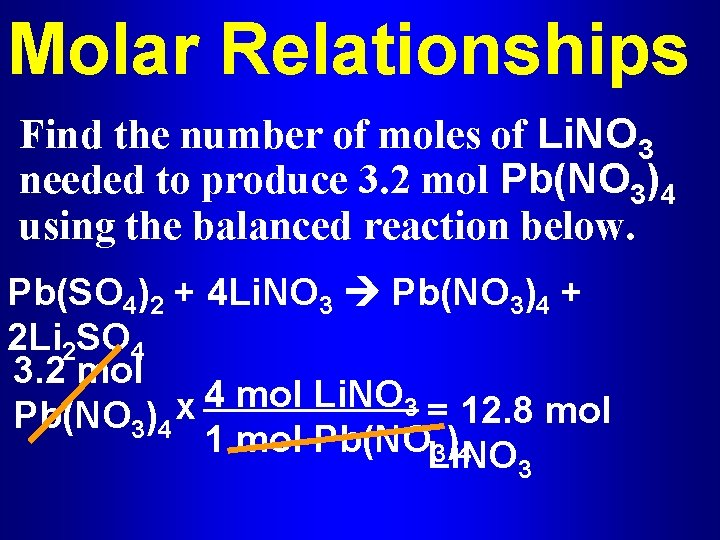Molar Relationships Find the number of moles of Li. NO 3 needed to produce 3. 2 mol Pb(NO 3)4 using the balanced reaction below. Pb(SO 4)2 + 4 Li. NO 3 Pb(NO 3)4 + 2 Li 2 SO 4 3. 2 mol 4 mol Li. NO 3 = 12. 8 mol x Pb(NO 3)4 1 mol Pb(NOLi. NO 3 )4 3Practice Problems Grab your clicker! (& a calculator)Question #1 Consider the balanced chemical reaction: 2 Fe + 3 H 2 S 04 → Fe 2(SO 4)3 + 3 H 2 How many moles of Fe are required to produce 9 moles of H 2? A. 9 moles B. 2 moles C. 4. 5 moles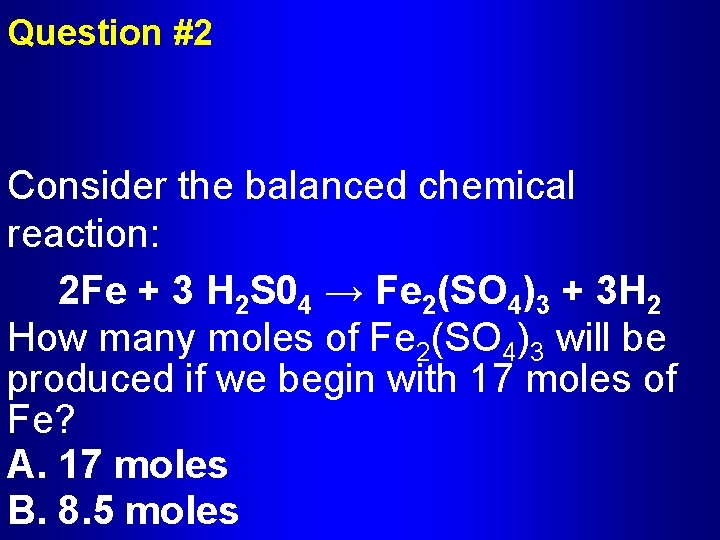Question #2 Consider the balanced chemical reaction: 2 Fe + 3 H 2 S 04 → Fe 2(SO 4)3 + 3 H 2 How many moles of Fe 2(SO 4)3 will be produced if we begin with 17 moles of Fe? A. 17 moles B. 8. 5 moles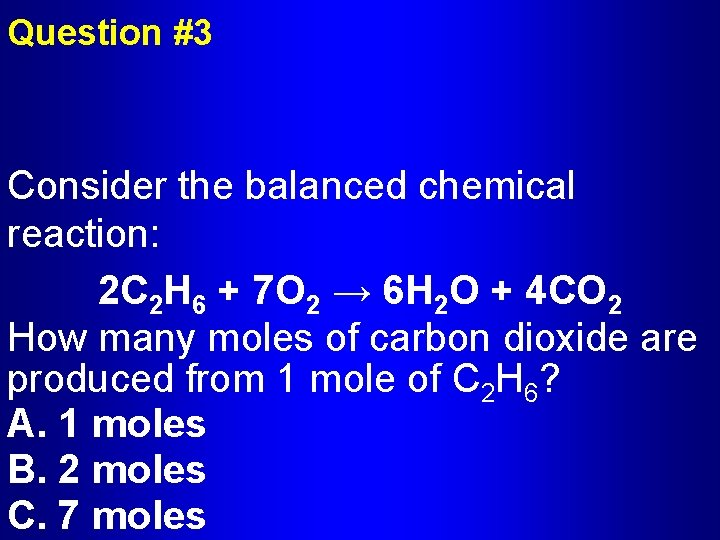Question #3 Consider the balanced chemical reaction: 2 C 2 H 6 + 7 O 2 → 6 H 2 O + 4 CO 2 How many moles of carbon dioxide are produced from 1 mole of C 2 H 6? A. 1 moles B. 2 moles C. 7 molesQuestion #4 Consider the balanced chemical reaction: 2 C 2 H 6 + 7 O 2 → 6 H 2 O + 4 CO 2 How many moles of O 2 are required to produce 9 moles of H 2 O? A. 10. 5 moles B. 6 moles C. 9 molesQuestion #5 Consider the following chemical reaction: KOH + H 3 PO 4→ K 3 PO 4 + H 2 O How many moles of KOH are required to produce 12. 5 moles of H 2 O? A. 2. 5 moles B. 7. 5 moles C. 9 moles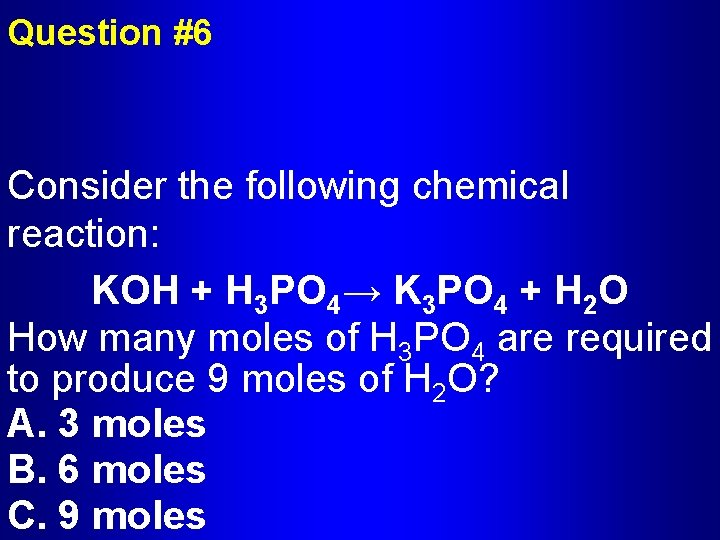Question #6 Consider the following chemical reaction: KOH + H 3 PO 4→ K 3 PO 4 + H 2 O How many moles of H 3 PO 4 are required to produce 9 moles of H 2 O? A. 3 moles B. 6 moles C. 9 moles